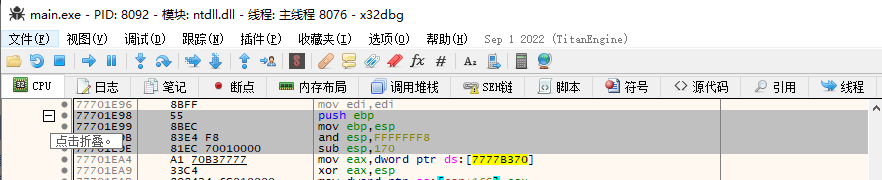﻿ 详解LyScript 内存扫描与查壳实现_python_脚本之家
python# 详解LyScript 内存扫描与查壳实现

## 正文

LyScript 中提供了多种内存特征扫描函数，每一种扫描函数用法各不相同，在使用扫描函数时应首先搞清楚他们之间的差异，如下将分别详细介绍每一种内存扫描函数是如何灵活运用的，最后将实现一个简易版内存查壳脚本，可快速定位目标程序加了什么壳。## 扫描ntdll.dll模块

```from LyScript32 import MyDebug
if __name__ == "__main__":
dbg = MyDebug()
conn = dbg.connect()
ref_one = dbg.scan_memory_one("55 8b ec 83 e4")
print("扫描一行: {}".format(hex(ref_one)))
ref_all = dbg.scan_memory_all("55 8b ec 83 e4")
for index in range(0, len(ref_all)):
print("记录: {} 地址: {}".format(index,hex(ref_all[index])))
dbg.close()
``````from LyScript32 import MyDebug
if __name__ == "__main__":
dbg = MyDebug()
conn = dbg.connect()
# 得到所有模块
local_module_base = dbg.get_all_module()
for index in local_module_base:
# 找到需要的模块
if index.get("name") == "msvcr120.dll":
entry = index.get("entry")
print("扫描入口: {}".format(hex(entry)))
# 切过去
dbg.set_register("eip",entry)
# 开始搜索特征
scan_ref = dbg.scan_memory_all("5d c2 0c 00 55 8b ec")
for x in scan_ref:
print("扫描到: {}".format(hex(x)))
dbg.close()
``````from LyScript32 import MyDebug
if __name__ == "__main__":
dbg = MyDebug()
conn = dbg.connect()
# 得到进程模块
local_module = dbg.get_all_module()
# 得到模块参数
module_base = local_module.get("base")
module_size = local_module.get("size")
print("基地址: {} 长度: {} 结束地址: {}".format(hex(module_base),hex(module_size),hex(module_base+module_size)))
# 扫描内存
ref = dbg.scan_memory_any(module_base,module_size,"51 5c a8 f8 4c 34 33")
if ref != False:
print("找到内存: {}".format(hex(ref)))
dbg.close()
```## 查壳功能

```from LyScript32 import MyDebug
# 查壳功能
def scan(dbg, string):
# 得到进程模块
local_module = dbg.get_all_module()
# 得到模块参数
module_base = local_module.get("base")
module_size = local_module.get("size")
# print("基地址: {} 长度: {} 结束地址: {}".format(hex(module_base),hex(module_size),hex(module_base+module_size)))
# 扫描内存
ref = dbg.scan_memory_any(module_base,module_size,string)
if ref != False:
return True
return False
if __name__ == "__main__":
dbg = MyDebug()
conn = dbg.connect()
# 存储特征码
signs = [
{"key": "Microsoft Visual C++ 2013", "value": "e8 ?? ?? ?? ?? e9 ?? ?? ?? ?? 55 8b ec"},
{"key": "UPX 3.96w", "value": "60 be ?? ?? ?? ?? 8d be 00 90 ff ff 57"}
]
for index in signs:
check = scan(dbg, index.get("value"))
if check == True:
print("编译特征： {}".format(index.get("key")))
dbg.close()
```

upx加壳软件输出为vs2013编译器特征输出